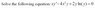# First-Order Linear ODE help?

decemberdays86
First-Order Linear ODE help?

This is my first post here. I'm still getting used to LATEX syntax so please forgive any mistakes. My question is on a simple differential equation... it doesn't appear to be exact or homogeneous... where do I start?

DD86

#### Attachments

•untitled.jpg
4.8 KB · Views: 375

Homework Helper
HINT: Look for an integrating factor.

decemberdays86
$$y'-4xy+2yln(y)/x=0$$

$$M(x,y)= -4xy \quad and \quad N(x,y)=2yln(y)/x$$

err.. I'm taking Calc 3 and diff eq at the same time so I hope my partials are right...

$$M_{y}=-4x \quad and \quad N_{x}=0$$

IntegFactor is defined as $$I(x) = e^{\int (M_{y} - N_{x})/N dx}$$ according to my notes. yeah I'm still stuck. Could someone verify the partial derivatives though?

EDIT: I now have $$y'+2y(-2x+ln(y)/x)=0$$ which looks nicer but I am still at a loss for what to do next...

What integrating factor am I looking for?

Last edited:
Homework Helper
December, need to put it into the form:

$$Mdx+Ndy=0$$

Thus for:

$$y^{'}-4xy+\frac{2yln(y)}{x}=0$$

we'd have:

$$(2yln(y)-4x^2y)dx+xdy=0$$

But that's not happening for me either. Can't see a way to solve it.

Tide . . . how about another hint? Somebody else too is ok.

Edit: Oh yea, it's not linear because of the ln(y) term.

Last edited:
Agnostic
DecemberDays86, This really doesn't have to do with anything, but, do you post on bodybuilding.com also?

decemberdays86
thanks for your input saltdog. My prof seems to like asking questions where you need to recognize "chain-ruled" things. For example, I have to get used to identifying a product of two terms as the derivative of some compossite function... not cool hehe

Homework Helper

Divide both sides of your equation by y, note that y'/y = (ln y)', multiply both sides by x and finally arrive at

$$\frac {d}{dx} \left( x^2 \ln y \right) =4 x^3$$

then see where you can go from there!

Homework Helper
Tide said:

Divide both sides of your equation by y, note that y'/y = (ln y)', multiply both sides by x and finally arrive at

$$\frac {d}{dx} \left( x^2 \ln y \right) =4 x^3$$

then see where you can go from there!

Ok, I get it now. Thanks Tide. You too December . . . PF rocks.decemberdays86
smart trick Tide!

here's my mess of a solution:

$$xy'+4x^2y-2ylny=0$$
$$y'-4xy=(-2ylny)/x$$

$$Let \quad y = e^{g(x)} \implies y'=g'e^{g(x)}$$

$$g'e^{g(x)}-4xe^{g(x)}= \frac {-2g(x)e^{g(x)}}{x}$$

divide out e^g(x) and rearrange

$$g'+\frac {2}{x}g=4x, Let \quad P(x) = 2/x \quad and \quad Q(x)=4x$$

$$g(x) = e^{- \int P(x) dx}[\int Q(x)e^{\int P(x) dx} dx + C]$$

$$\frac {1}{x^2}[x^4+C] \implies g(x)=x^2 + \frac {C}{x^2}$$

QUESTION: am I allowed to call the "c/x^2" term just another constant?

back-sub $$y(x) = e^{g(x)} = e^{(x^2)}*e^{\frac {C}{x^2}}$$

Thanks for all of your help.

Last edited: